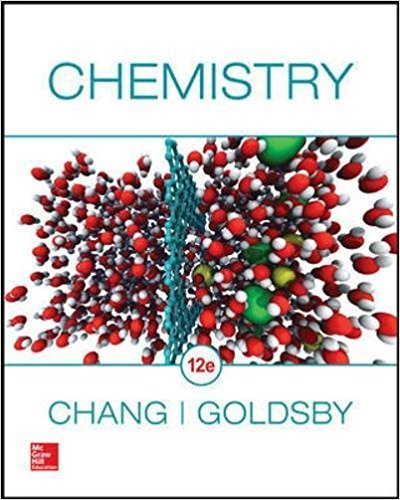×

×

# Solutions for Chapter 1: Chemistry: The Study of Change## Full solutions for Chemistry | 12th Edition

ISBN: 9780078021510Solutions for Chapter 1: Chemistry: The Study of Change

Solutions for Chapter 1
4 5 0 304 Reviews
23
0
##### ISBN: 9780078021510

This textbook survival guide was created for the textbook: Chemistry, edition: 12. This expansive textbook survival guide covers the following chapters and their solutions. Since 115 problems in chapter 1: Chemistry: The Study of Change have been answered, more than 138018 students have viewed full step-by-step solutions from this chapter. Chapter 1: Chemistry: The Study of Change includes 115 full step-by-step solutions. Chemistry was written by and is associated to the ISBN: 9780078021510.

Key Chemistry Terms and definitions covered in this textbook
• Alditol

The product formed when the C"O group of a monosaccharide is reduced to a CHOH group.

• alkoxymercuration-demercuration

A two-step process that achieves Markovnikov addition of an alcohol (H and OR) across an alkene. The product of this process is an ether.

• Alkyne

An unsaturated hydrocarbon that contains one or more carbon-carbon triple bonds.

• Arene

A term used to classify benzene and its derivatives.

• Benzylic position

An sp3 -hybridized carbon bonded to a benzene ring

• cation

A structure that bears a positive charge.

• chemical equilibrium.

A state in which the rates of the forward and reverse reactions are equal. (14.1)

A reaction in which two reactants add together in a single step to form a cyclic product. The best known of these is the Diels-Alder reaction

• frontier orbital theory

The analysis of a reaction using MO theory, where only the frontier orbitals (HOMO and LUMO) are considered.

• Henderson-Hasselbalch equation

An equation that is often employed to calculate the pH of buffered solutions: pH = pKa + log 3conjugated base4 3acid4

• ion

Electrically charged atom or group of atoms (polyatomic ion); ions can be positively or negatively charged, depending on whether electrons are lost (positive) or gained (negative) by the atoms. (Section 2.7)

• ionic reaction

A reaction that involves the participation of ions as reactants, intermediates, or products.

• Pauli exclusion principle

No more than two electrons may be present in an orbital. If two electrons are present, their spins must be paired

• resolution

The separation of enantiomers from a mixture containing both enantiomers.

• reversible process

A process that can go back and forth between states along exactly the same path; a system at equilibrium is reversible if equilibrium can be shifted by an infinitesimal modification of a variable such as temperature. (Section 19.1)

• SN2

A bimolecular nucleophilic substitution reaction.

• superimposable

Two objects that are identical.

• Twist-boat conformation

A nonplanar conformation of a cyclohexane ring that is twisted from and slightly more stable than a boat conformation.

• Unimolecular reaction

A reaction in which only one species is involved in the rate-determining step

• vibrational excitation

In IR spectroscopy, the energy of a photon is absorbed and temporarily stored as vibrational energy